Search by Topic

Resources tagged with Triangles similar to Inside Triangles:

Filter by: Content type:
Age range:
Challenge level:

There are 62 results

Broad Topics > Angles, Polygons, and Geometrical Proof > Triangles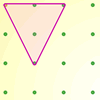Inside Triangles

Age 5 to 7 Challenge Level:

How many different triangles can you draw on the dotty grid which each have one dot in the middle?Nine-pin Triangles

Age 7 to 11 Challenge Level:

How many different triangles can you make on a circular pegboard that has nine pegs?Triangles All Around

Age 7 to 11 Challenge Level:

Can you find all the different triangles on these peg boards, and find their angles?Tri.'s

Age 7 to 11 Challenge Level:

How many triangles can you make on the 3 by 3 pegboard?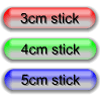Triangle Edges

Age 5 to 7 Challenge Level:

How many triangles can you make using sticks that are 3cm, 4cm and 5cm long?Triangle Pin-down

Age 7 to 11 Challenge Level:

Use the interactivity to investigate what kinds of triangles can be drawn on peg boards with different numbers of pegs.More Transformations on a Pegboard

Age 7 to 11 Challenge Level:

Use the interactivity to find all the different right-angled triangles you can make by just moving one corner of the starting triangle.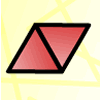Triangle Animals

Age 5 to 7 Challenge Level:

How many different ways can you find to join three equilateral triangles together? Can you convince us that you have found them all?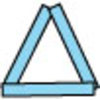Sticks and Triangles

Age 7 to 11 Challenge Level:

Using different numbers of sticks, how many different triangles are you able to make? Can you make any rules about the numbers of sticks that make the most triangles?Putting Two and Two Together

Age 7 to 11 Challenge Level:

In how many ways can you fit two of these yellow triangles together? Can you predict the number of ways two blue triangles can be fitted together?Egyptian Rope

Age 7 to 11 Challenge Level:

The ancient Egyptians were said to make right-angled triangles using a rope with twelve equal sections divided by knots. What other triangles could you make if you had a rope like this?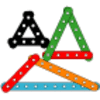Let's Investigate Triangles

Age 5 to 7 Challenge Level:

Vincent and Tara are making triangles with the class construction set. They have a pile of strips of different lengths. How many different triangles can they make?Uncanny Triangles

Age 7 to 11 Challenge Level:

Can you help the children find the two triangles which have the lengths of two sides numerically equal to their areas?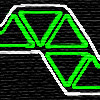Tri-five

Age 7 to 11 Challenge Level:

Find all the different shapes that can be made by joining five equilateral triangles edge to edge.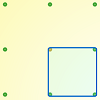Peg and Pin Boards

Age 5 to 11

This article for teachers suggests activities based on pegboards, from pattern generation to finding all possible triangles, for example.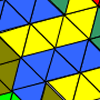Tessellating Transformations

Age 7 to 11 Challenge Level:

Can you find out how the 6-triangle shape is transformed in these tessellations? Will the tessellations go on for ever? Why or why not?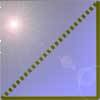Cutting Corners

Age 7 to 11 Challenge Level:

Can you make the most extraordinary, the most amazing, the most unusual patterns/designs from these triangles which are made in a special way?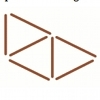Seven Sticks

Age 5 to 7 Challenge Level:

Explore the triangles that can be made with seven sticks of the same length.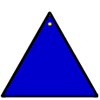Posting Triangles

Age 5 to 7 Challenge Level:

If you can post the triangle with either the blue or yellow colour face up, how many ways can it be posted altogether?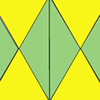Repeating Patterns

Age 5 to 7 Challenge Level:

Try continuing these patterns made from triangles. Can you create your own repeating pattern?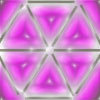Six to Four

Age 5 to 7 Challenge Level:

Move four sticks so there are exactly four triangles.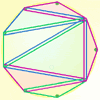Board Block

Age 5 to 7 Challenge Level:

Take it in turns to make a triangle on the pegboard. Can you block your opponent?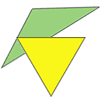Triangle Relations

Age 7 to 11 Challenge Level:

What do these two triangles have in common? How are they related?Matching Triangles

Age 5 to 7 Challenge Level:

Can you sort these triangles into three different families and explain how you did it?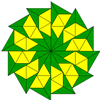Triangle Shapes

Age 5 to 11 Challenge Level:

This practical problem challenges you to create shapes and patterns with two different types of triangle. You could even try overlapping them.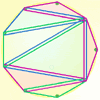Board Block for Two

Age 5 to 7 Challenge Level:

Board Block game for two. Can you stop your partner from being able to make a shape on the board?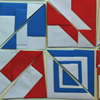Paper Patchwork 2

Age 5 to 7 Challenge Level:

Have a go at making a few of these shapes from paper in different sizes. What patterns can you create?Triangle or No Triangle?

Age 5 to 7 Challenge Level:

Here is a selection of different shapes. Can you work out which ones are triangles, and why?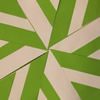Folding Flowers 2

Age 7 to 11 Challenge Level:

Make a flower design using the same shape made out of different sizes of paper.Cutting it Out

Age 5 to 11 Challenge Level:

I cut this square into two different shapes. What can you say about the relationship between them?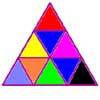Colouring Triangles

Age 5 to 7 Challenge Level:

Explore ways of colouring this set of triangles. Can you make symmetrical patterns?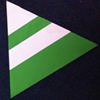Regular Rings 1

Age 7 to 11 Challenge Level:

Can you work out what shape is made by folding in this way? Why not create some patterns using this shape but in different sizes?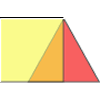Overlaps

Age 5 to 7 Challenge Level:

What does the overlap of these two shapes look like? Try picturing it in your head and then use the interactivity to test your prediction.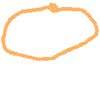Three Fingers and a Loop of String

Age 5 to 7 Challenge Level:

Using a loop of string stretched around three of your fingers, what different triangles can you make? Draw them and sort them into groups.Animated Triangles

Age 5 to 7 Challenge Level:

Watch this "Notes on a Triangle" film. Can you recreate parts of the film using cut-out triangles?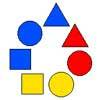Chain of Changes

Age 5 to 7 Challenge Level:

Arrange the shapes in a line so that you change either colour or shape in the next piece along. Can you find several ways to start with a blue triangle and end with a red circle?Cut it Out

Age 7 to 11 Challenge Level:

Can you dissect an equilateral triangle into 6 smaller ones? What number of smaller equilateral triangles is it NOT possible to dissect a larger equilateral triangle into?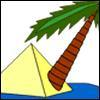Triangle Island

Age 7 to 11 Challenge Level:

You have pitched your tent (the red triangle) on an island. Can you move it to the position shown by the purple triangle making sure you obey the rules?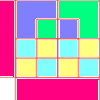Diagrams

Age 7 to 11 Challenge Level:

A group activity using visualisation of squares and triangles.Data Shapes

Age 5 to 7 Challenge Level:

Sara and Will were sorting some pictures of shapes on cards. "I'll collect the circles," said Sara. "I'll take the red ones," answered Will. Can you see any cards they would both want?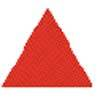Tessellating Triangles

Age 7 to 11 Challenge Level:

Can you make these equilateral triangles fit together to cover the paper without any gaps between them? Can you tessellate isosceles triangles?Cut and Make

Age 7 to 11 Challenge Level:

Cut a square of paper into three pieces as shown. Now,can you use the 3 pieces to make a large triangle, a parallelogram and the square again?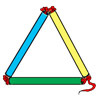Making Maths: Test the Strength of a Triangle

Age 7 to 11 Challenge Level:

Have you noticed that triangles are used in manmade structures? Perhaps there is a good reason for this? 'Test a Triangle' and see how rigid triangles are.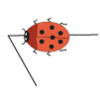Walking Round a Triangle

Age 5 to 7 Challenge Level:

This ladybird is taking a walk round a triangle. Can you see how much he has turned when he gets back to where he started?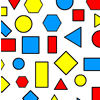Sorting Logic Blocks

Age 5 to 11 Challenge Level:

This interactivity allows you to sort logic blocks by dragging their images.Name That Triangle!

Age 7 to 11 Challenge Level:

Can you sketch triangles that fit in the cells in this grid? Which ones are impossible? How do you know?Jig Shapes

Age 5 to 11 Challenge Level:

Can you each work out what shape you have part of on your card? What will the rest of it look like?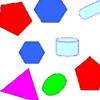Part the Polygons

Age 7 to 11 Short Challenge Level:

Draw three straight lines to separate these shapes into four groups - each group must contain one of each shape.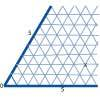Cartesian Isometric

Age 7 to 11 Challenge Level:

The graph below is an oblique coordinate system based on 60 degree angles. It was drawn on isometric paper. What kinds of triangles do these points form?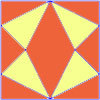Fraction Fascination

Age 7 to 11 Challenge Level:

This problem challenges you to work out what fraction of the whole area of these pictures is taken up by various shapes.